Problem-solving in mathematics helps children develop reasoning and communication skills that are transferrable and important life skills.

Reasoning is required on three levels when children solve word problems. First, they use reasoning to recognise what information is provided or missing.

Then, they use reasoning to figure out what information they need to find. Finally, they use reasoning to draw on relevant prior knowledge and choose the most suitable heuristic to work out the solution.

Communication is required for comprehension and expression during problem-solving. Children need to read and understand word problems and then write and express their solutions.

When children are encouraged to explain their thinking verbally, visually and in written form, they gain a better understanding of math concepts and develop stronger communication skills.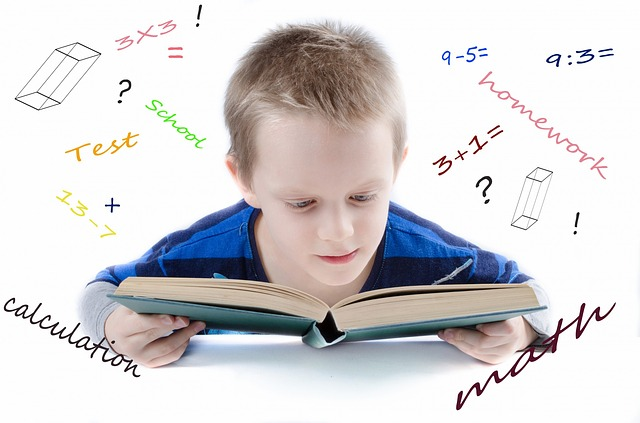Singapore Math Heuristics

In part one of our Math Heuristics series, we gave an overview of the 12 heuristics in Singapore Primary Math syllabus, with tips from the curriculum team at Seriously Addictive Mathematics (S.A.M) on how to solve various math word problems using them.

In part two of the Math Heuristics series, we expanded on the heuristics – Act It Out, Draw A Diagram and Look For Patterns, and also demonstrated how to apply the Polya’s 4-step problem-solving process in sample word problems.

In the third part of the Math Heuristics series, we focused on the heuristics: Make a Systematic List, Guess and Check and Restate the Problem in Another Way.

In part 4 of this series, we will zoom in on these 3 heuristics: Solve Part of the Problem, Simplify the Problem and Work Backwards.

Heuristic: Solve Part of the Problem

Word Problem (Primary 3):

At a school library, each student could borrow up to 4 books. The bar graph below shows how many books students borrowed from the school library in one week. What was the total number of books borrowed from the library that week?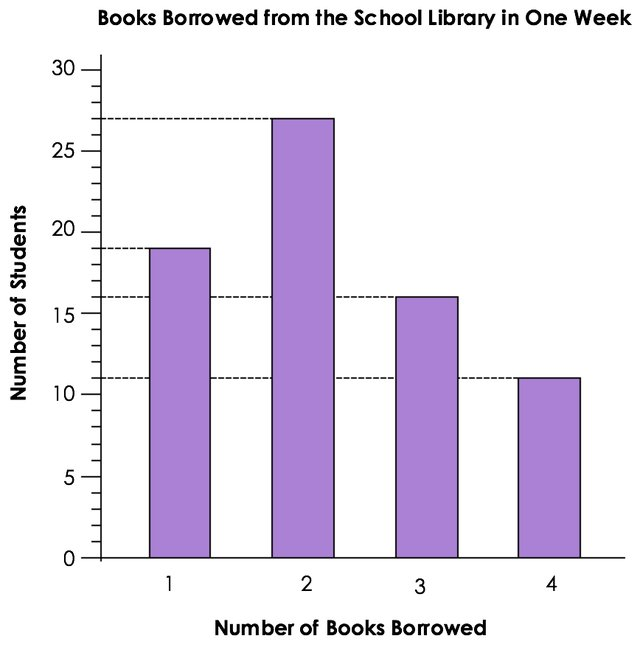Solution:

1. Understand:
What to find: total number of books borrowed
What is known: 19 students borrowed 1 book. 27 students borrowed 2 books. 16 students borrowed 3 books. 11 students borrowed 4 books.

2. Choose: Solve part of the problem

3. Solve:

19 students borrowed 1 book = 19 × 1 = 19 books
27 students borrowed 2 books = 27 × 2 = 54 books
16 students borrowed 3 books = 16 × 3 = 48 books
11 students borrowed 4 books = 11 × 4 = 44 books

19 + 54 + 48 + 44 = 165 books

165 books were borrowed from the library that week.

4. Check:
Did I read the information from the bar graph correctly? Yes
Did I calculate the number of books for each bar correctly? Yes
Did I add the number of books for all bars? Yes

Try solving the following word problem using Polya’s 4-step process.

Word Problem (Primary 6):

The diagram below shows 4 shaded triangles in Triangle ABC. All the triangles in the diagram are equilateral triangles. If the area of Triangle ABC is 64 cm2, find the total area of the shaded triangles.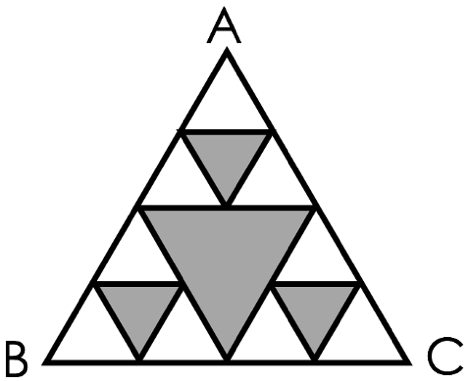Answer: The total area of the shaded triangles is 28 cm2.
See the solution in part one of our Singapore Math Heuristics series.

Heuristic: Simplify the Problem

Word Problem (Primary 3):

A bakery sold cupcakes at \$4 each. For every 6 cupcakes bought, a discount of \$2 was given. Ling bought 50 cupcakes. How much did Ling pay for the cupcakes?Solution:

1. Understand:
What to find: How much Ling paid for the cupcakes.
What is known: Each cupcake cost \$4. A discount of \$2 was given for every 6 cupcakes bought. Ling bought 50 cupcakes.

2. Choose: Simplify the problem

3. Solve:

6 cupcakes form 1 set.
50 ÷ 6 = 8 remainder 2
Ling bought 8 sets of 6 cupcakes and 2 more cupcakes.

Each cupcake costs \$4.
6 × \$4 = \$24
\$24 – \$2 = \$22.
Each set of 6 cupcakes cost \$22.

Cost of 8 sets of 6 cupcakes = 8 × \$22 = \$176
Cost of 2 more cupcakes = 2 × \$4 = \$8
Total cost = \$176 + \$8 = \$184

Ling paid \$184 for the cupcakes.

4. Check:
How many cupcakes are there in 8 sets? 8 × 6 = 48
Were there 50 cupcakes? 48 + 2 = 50. Yes

Word Problem (Primary 4):

Square EFGH is made up of 4 rectangles. The perimeter of Square EFGH is 32 centimetres. Find the perimeter of each rectangle.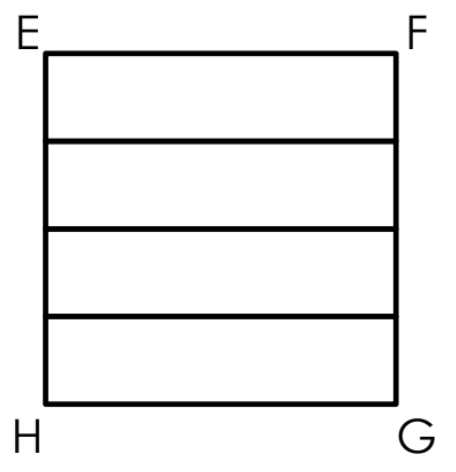Solution:

1. Understand:
What to find: The perimeter of each rectangle.
What is known: Square EFGH is made up of 4 rectangles. The perimeter of EFGH is 32cm. EF is made up of the length of a rectangle. FG is made up of the breadths of 4 rectangles.

2. Choose: Simplify the problem

3. Solve:

A square has 4 equal sides.
32 ÷ 4 = 8
EF is 8cm. The length of a rectangle is 8cm.
FG is also 8cm.
8 ÷ 4 = 2
The breadth of a rectangle is 2cm.

8 + 2 + 8 + 2 = 20
The perimeter of each rectangle is 20 centimetres.

4. Check:
Is the perimeter of EFGH 32cm?
8 + 2 + 2 + 2 + 2 + 8 + 2 + 2 + 2 + 2 = 32. Yes.

Is EF the same length as FG?
EF = 8. FG = 4 × 2 = 8. Yes.

Try solving the following word problem using Polya’s 4-step process.

Word Problem (Primary 6):

The shaded figure below shows a semicircle and two quarter circles. Find the area of the shaded figure. (Take pi = 3.14)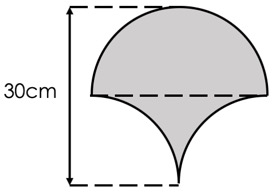Answer: The area of the shaded figure is 450 cm2.
See the solution in part one of our Singapore Math Heuristics series.

Heuristic: Work Backwards

Word Problem (Primary 3):

Mr Adam had some pens in his stationery store. He sold 318 of the pens at \$1 each. He then put the remaining pens into packs of 5 and sold each pack for \$3. He made \$249 from selling all the packs. How many pens did Mr Adam have at first?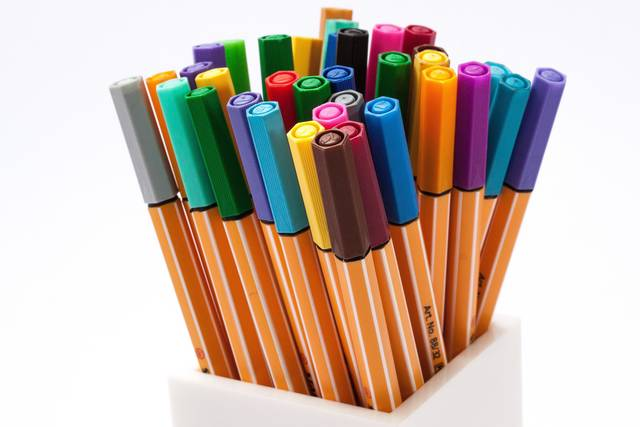Solution:

1. Understand:
What to find: How many pens did Mr Adam have at first?
What is known: He sold 318 pens. He packed the remaining pens into packs of 5. He sold the packs at \$3 each and made \$249.

2. Choose: Work backwards

3. Solve:

Let’s label the changes C1, C2 and C3
C1: He sold 318 pens.
C2: He packed the remaining pens into packs of 5
C3: He sold the packs at \$3 each.

We can organise the information like this: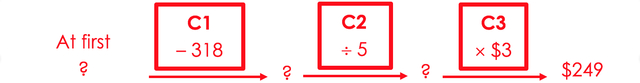C3 is he sold the packs at \$3 each.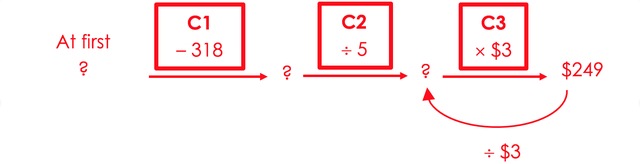Before C3: 249 ÷ 3 = 83
He had 83 packs.

C2 is he packed the remaining pens into packs of 5.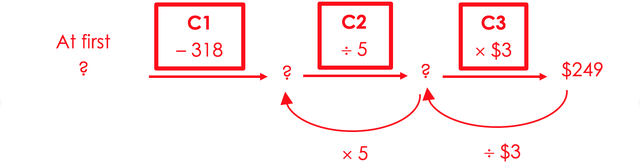Before C2: 83 × 5 = 415
He had 415 pens remaining.

C1 is he sold 318 pens.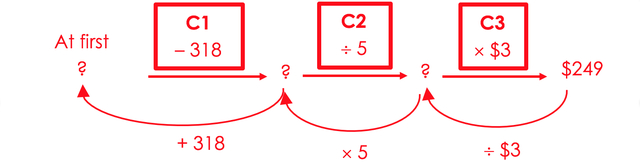Before C1: 415 + 318 = 733
Mr Adam had 733 pens at first.

4. Check:
How many remaining pens did he have? 733 – 318 = 415
How many packs of 5 pens did he have? 415 ÷ 5 = 83
How much did he made from selling the packs? 83 × 3 = \$249

Try solving the following word problem using Polya’s 4-step process.

Word Problem (Primary 3):

Darren had some stickers in his collection. He bought 20 more stickers and gave 33 stickers to his sister. He had 46 stickers left. How many stickers did Darren have in his collection at first?

Answer: Darren has 59 stickers in his collection at first.
See the solution in part one of our Singapore Math Heuristics series.

Look out for the final part of this series for the remaining Math Heuristics and word problems with step-by-step worked solutions.

This is the fourth part to S.A.M Math Heuristics series. Read part one, part two and part three here.

Established in 2010, Seriously Addictive Mathematics (S.A.M) is the world’s largest Singapore Math enrichment program for children aged four to 12. The award-winning S.A.M program is based on the global top-ranking Singapore Math curriculum with a focus on developing problem solving and thinking skills.

The curriculum is complemented with S.A.M’s two-pillared approach of Classroom Engagement and Worksheet Reinforcement, with an individual learning plan tailored to each child at their own skill level and pace, because no two children learn alike.

This post is brought to you by Seriously Addictive Mathematics.Like what you see here? Get parenting tips and stories straight to your inbox! Join our mailing list here.

Want to be heard and seen by over 100,000 parents in Singapore? We can help! Leave your contact here and we’ll be in touch.